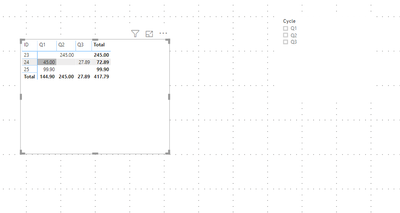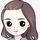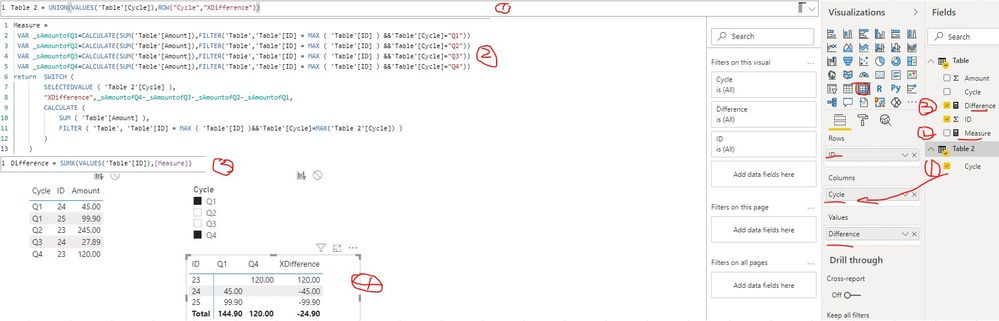cancel
Showing results for
Did you mean:Helper III

## Looking for difference between two values by filters

Hi All,

I am looking for difference between two values like q2 and a3 cycles result should display the difference values based on the filter selected.

below the example: when i select the q2 and q3 it should display difference between those values at the end.1 ACCEPTED SOLUTIONCommunity Support

Hi @Naveen_SV ,

I created a sample pbix file (see attachment) for you, please check whether that is what you want.

1. Create a calculated table: Table 2

``Table 2 = UNION(VALUES('Table'[Cycle]),ROW("Cycle","XDifference"))``

2. Create measures to get the sum amount and difference

``````Measure =
VAR _sAmountofQ1=CALCULATE(SUM('Table'[Amount]),FILTER('Table','Table'[ID] = MAX ( 'Table'[ID] ) &&'Table'[Cycle]="Q1"))
VAR _sAmountofQ2=CALCULATE(SUM('Table'[Amount]),FILTER('Table','Table'[ID] = MAX ( 'Table'[ID] ) &&'Table'[Cycle]="Q2"))
VAR _sAmountofQ3=CALCULATE(SUM('Table'[Amount]),FILTER('Table','Table'[ID] = MAX ( 'Table'[ID] ) &&'Table'[Cycle]="Q3"))
VAR _sAmountofQ4=CALCULATE(SUM('Table'[Amount]),FILTER('Table','Table'[ID] = MAX ( 'Table'[ID] ) &&'Table'[Cycle]="Q4"))
return  SWITCH (
SELECTEDVALUE ( 'Table 2'[Cycle] ),
"XDifference",_sAmountofQ4-_sAmountofQ3-_sAmountofQ2-_sAmountofQ1,
CALCULATE (
SUM ( 'Table'[Amount] ),
FILTER ( 'Table', 'Table'[ID] = MAX ( 'Table'[ID] )&&'Table'[Cycle]=MAX('Table 2'[Cycle]) )
)
) ``````
``Difference = SUMX(VALUES('Table'[ID]),[Measure]) ``

3. Create a matrix visual (Rows: ID   Column: field Cycle of Table 2  Values: [Difference] )Best Regards
Rena
Community Support Team _ Rena Ruan
If this post helps, then please consider Accept it as the solution to help the other members find it more.

Community Support Team _ Rena
If this post helps, then please consider Accept it as the solution to help the other members find it more quickly.
6 REPLIES 6Helper IICommunity Support

Hi @Naveen_SV ,

What's your expected result? Whether the below table is what you want? If yes, you can refer the following threads to get it.

 ID Q1 Q2 Q3 difference 23 245 245 24 45 27.89 -17.11 25 99.9 99.9

Subtract values in a measure for different categories

Dax - how to subtract one pivoted matrix column from another (power bi)

Best Regards

Rena

Community Support Team _ Rena Ruan
If this post helps, then please consider Accept it as the solution to help the other members find it more.

Community Support Team _ Rena
If this post helps, then please consider Accept it as the solution to help the other members find it more quickly.Helper III

@v-yiruan-msft  Thanks for your reply, where here the scenrio is q1, q2, q3 is dynamicCommunity Support

Hi @Naveen_SV ,

I created a sample pbix file (see attachment) for you, please check whether that is what you want.

1. Create a calculated table: Table 2

``Table 2 = UNION(VALUES('Table'[Cycle]),ROW("Cycle","XDifference"))``

2. Create measures to get the sum amount and difference

``````Measure =
VAR _sAmountofQ1=CALCULATE(SUM('Table'[Amount]),FILTER('Table','Table'[ID] = MAX ( 'Table'[ID] ) &&'Table'[Cycle]="Q1"))
VAR _sAmountofQ2=CALCULATE(SUM('Table'[Amount]),FILTER('Table','Table'[ID] = MAX ( 'Table'[ID] ) &&'Table'[Cycle]="Q2"))
VAR _sAmountofQ3=CALCULATE(SUM('Table'[Amount]),FILTER('Table','Table'[ID] = MAX ( 'Table'[ID] ) &&'Table'[Cycle]="Q3"))
VAR _sAmountofQ4=CALCULATE(SUM('Table'[Amount]),FILTER('Table','Table'[ID] = MAX ( 'Table'[ID] ) &&'Table'[Cycle]="Q4"))
return  SWITCH (
SELECTEDVALUE ( 'Table 2'[Cycle] ),
"XDifference",_sAmountofQ4-_sAmountofQ3-_sAmountofQ2-_sAmountofQ1,
CALCULATE (
SUM ( 'Table'[Amount] ),
FILTER ( 'Table', 'Table'[ID] = MAX ( 'Table'[ID] )&&'Table'[Cycle]=MAX('Table 2'[Cycle]) )
)
) ``````
``Difference = SUMX(VALUES('Table'[ID]),[Measure]) ``

3. Create a matrix visual (Rows: ID   Column: field Cycle of Table 2  Values: [Difference] )Best Regards
Rena
Community Support Team _ Rena Ruan
If this post helps, then please consider Accept it as the solution to help the other members find it more.

Community Support Team _ Rena
If this post helps, then please consider Accept it as the solution to help the other members find it more quickly.Helper II

Hi , Great answer. I have different query regarding this.

you are doing Q4 as base (q4-q3-q2-q1). If I'm selecting the filter Q3 & Q2 ,it should take Q3 is base (I need answer like Q3 - Q2 ) not like (-q3-q2).

same way for ,if  I'm selecting q2 and q1 in filter , it should show q2-q1 (not like -q2-q1).Helper III

@v-yiruan-msft  thanks it worked